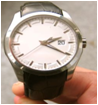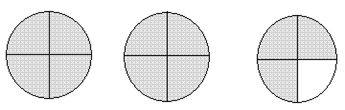# Indian Talent Olympiad - IMO PDF Sample Papers for Class 3

Class 3 sample paper & practice questions for International Maths Olympiad (IMO) level 1 are given below. Syllabus for level 1 is also mentioned for these exams. You can refer these sample paper & quiz for preparing for the exam.#### Resources:

##### Sample Questions from Olympiad Success:
 Q.1 Q.2 Q.3 Q.4 Q.5 Q.6 Q.7 Q.8 Q.9 Q.10
 Q.1 How many sevens are there in 735? (IMO L2 2012) a) 15 b) 105 c) 17 d) 150
 Q.2 Sunny and Poonam have 9,850 paise and 4,523 paise respectively. Alia has an amount equal to both of them, then how much Rs. does Alia have? a) Rs. 143.73 b) Rs. 14.37 c) Rs. 134.73 d) None of the above
 Q.3 Find the difference between the largest possible even number and smallest possible odd number from given digits:6, 5, 8, 9, 3.   (IMO L2 2014) a) 62,847 b) 62,964 c) 62,874 d) 62,946
 Q.4 Which of the following options has the smallest value? a) 4 + 3 + 2 + 1 b) 4 + 3 + 2 x 1 c) 4 + 3 x 2 + 1 d) 4 x 3 + 2 + 1
 Q.5 What time will the analog wristwatch show in 45 minutes?a) 15:45 b) 2:65 c) 3:05 d) 2:45
 Q.6 Arrange these numbers from the greatest to the smallest:56, 3 × 8, 29, 2 × 7, 2, 19.   (IMO L2 2012) a) 56, 2 × 7, 3 × 8, 29, 2, 19. b) 2, 2 × 7, 19, 3 × 8, 29, 56. c) 56, 29, 3 × 8, 19, 2 × 7, 2. d) 2 × 7, 3 × 8, 29, 2, 19, 56.
 Q.7 If one well contains 45 L 123 mL of water and the other well contains 56 L 452 mL of water, then what is the total quantity of water needed to balance the wells? a) 28 L 952 mL b) 36 L 754 mL c) 10 L 587 mL d) 11 L 329 mL
 Q.8 How many 2-digit numbers can you make using 1 and 2(digits can be used more than 1)? a) 6 b) 3 c) 5 d) 4
 Q.9 What fraction is the shaded part?a) 2 b) 2 1/4 c) 1 3/4 d) 2 3/4
 Q.10 Which among the following is less and by how much? (75.16 + 12.2) or (500.12 - 412.78) a) (500.12 - 412.78) is greater and the difference is Rs. 0.2 b) (500.12 - 412.78) is greater and the difference is 2 paise c) (75.16 + 12.2) is greater and the difference is Rs. 0.2 d) (75.16 + 12.2) is greater and the difference is 2 paiseSample PDF of Indian Talent Olympiad - International Maths Olympiad (IMO) PDF Sample Papers for Class 3:

 Q.1 )b Q.2 )a Q.3 )a Q.4 )b Q.5 )c Q.6 )c Q.7 )d Q.8 )d Q.9 )d Q.10 )d

Q.1 : b | Q.2 : a | Q.3 : a | Q.4 : b | Q.5 : c | Q.6 : c | Q.7 : d | Q.8 : d | Q.9 : d | Q.10 : d